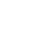0

# Thales’s Theorem

For the theorem sometimes called Thales’ theorem and pertaining to similar triangles, see intercept theorem.

In geometryThales’s theorem states that if A, B, and C are distinct points on a circle where the line AC is a diameter, the angle ABC is a right angle. Thales’s theorem is a special case of the inscribed angle theorem and is mentioned and proved as part of the 31st proposition in the third book of Euclid‘s ElementsIt is generally attributed to Thales of Miletus but it is sometimes attributed to Pythagoras.  ( Learn more )

Thales’s Theorem is a special case of the inscribed angle theorem:

In geometry, an inscribed angle is the angle formed in the interior of a circle when two chords intersect on the circle. It can also be defined as the angle subtended at a point on the circle by two given points on the circle.

Equivalently, an inscribed angle is defined by two chords of the circle sharing an endpoint.

The inscribed angle theorem (Also known as Star Trek Lemma) relates the measure of an inscribed angle to that of the central angle subtending the same arc. ( Learn more )

## Like it? Share with your friends!

0hate
0
hateconfused
0
confusedfail
0
failfun
0
fungeeky
0
geekylove
0
lovelol
0
lolomg
0
omgwin
0
win